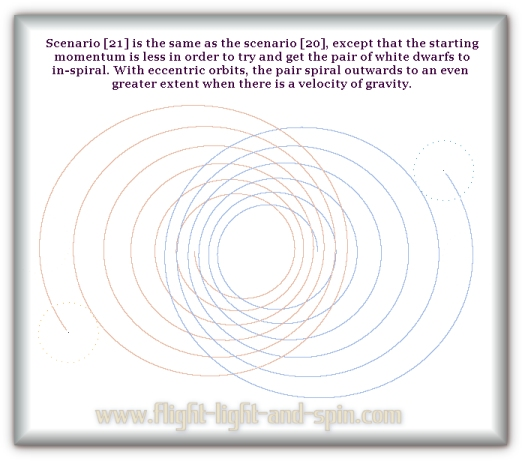A White Dwarf binary system with gravity velocity

 1 2 3 4 5 6 7 8 9 10
 6 The scale of OGS11 is pre-set to the LIGO data; so BOGVOS has not been explored on a more typical stellar scale. Scenarios - were constructed which use 1-solar-mass white-dwarf stars; to see if this lesser mass (and corresponding lesser velocity/gravity) would affect BOGVOS (Binary-Orbit Gravity-Velocity Out-Spiral). Due to their lower velocity, the white-dwarfs therefore have a much smaller difference between the gravity origin and the position of the bodies; only a 40 km difference as opposed to about 240km for the LIGO pair at about 2300km apart. And yet, the white dwarfs spiral outwards anyway. However, they did out-spiral to a lesser degree than the LIGO pair.Scenario : The white-dwarfs start 2457km apart. At each orbit the maximum distance from each other increases by an amount that is itself increasing. Conservatively: At each orbit of the outwards spiral they are increasing the rate of separation by over 50km. So after 1000 orbits, this rate of increase is at least 50 000km more for each orbit. So the average increase in the rate of separation is half of that: 25 000km increase per orbit. So after 1000 orbits they are over 25 million km apart. Now let us run this approximation for 20 000 orbits. After 20 000 they are increasing their rate of separation by a million km per orbit (20 000 x 50km). So that is an average of half a million km increase per orbit for the 20 000 orbits. So at this point they are separated by a distance several times that of the Sun to Pluto. I see every reason, inductively speaking, that this amount should continue to increase if the pair had an orbital year the same as the Alpha Centauri binary of 80 Earth-years. Using these rough conservative approximations please try and appreciate how this would be contradictory to normal binary pairs having a life-span of more than a very small amount in astronomical terms – if gravity travels at the velocity of light. My rough estimate is that perhaps after 10 thousand years to 100 thousand years, all stellar binaries would no longer have significant gravitational force to hold together as a pair due to BOGVOS – if and only if – gravity traveled at the velocity of light as Einstein’s Theory of General Relativity would have us accept. The Newtonian paradigm results in no such problems. Observed estimates of the number of binary pairs vary upwards from half the known stars in the Galaxy. It is ridiculous to conclude that they all split in half in comparatively recent observed times of thousands of years. So I feel reasonably certain, (simply on the basis of these rough estimates) that gravity operating at the velocity of light is inconsistent with the Universe as we find it. But this conclusion is based on estimates in advance of the full computation. In OGS12 I will be able to give more accurate numbers regarding the time it takes for a binary pair to reach the same distance apart as the Alpha Centauri binary – if gravity operates at the velocity of light. .
 We also need to consider starting the white-dwarf pair with much less momentum. The graphic following is for scenario . Starting with less momentum did not lessen the rate of out-spiraling. In fact, the rate of rate-increase is itself increasing here.Just based on observing the graph above, it seems that the more eccentric the orbit, the greater the outwards spiral. The eccentricity is the result of less starting momentum. So the earlier estimates for the previous example have not taken into account that the rate of rate-increase is itself increasing. You can see for yourself by observing scenario  and then gauge some estimates for the life-span of the highly eccentric Alpha Centauri binary pair – if we have velocity to gravity. Surely the Alpha Centauri pair should out-spiral due to the gravity origin being behind the position of the body? For now the question reduces to one of scale however, so I make no outright predictions until OGS12 has been constructed and tested thoroughly. The white-dwarfs in these graphics are still on the scale of the LIGO data. They are only a few thousand kilometers apart at most – with each orbit taking just seconds. .

 Next Page ->

^ Top of Page ^